New to Qlik Sense

If you’re new to Qlik Sense, start with this Discussion Board and get up-to-speed quickly.

Announcements
QLIKWORLD LIVE! MAY 16 - 19TH, EARLY BIRD DISCOUNTS! REGISTER TODAY
cancel
Showing results for
Did you mean: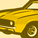Contributor

COUNT multiple specific values with multiple conditions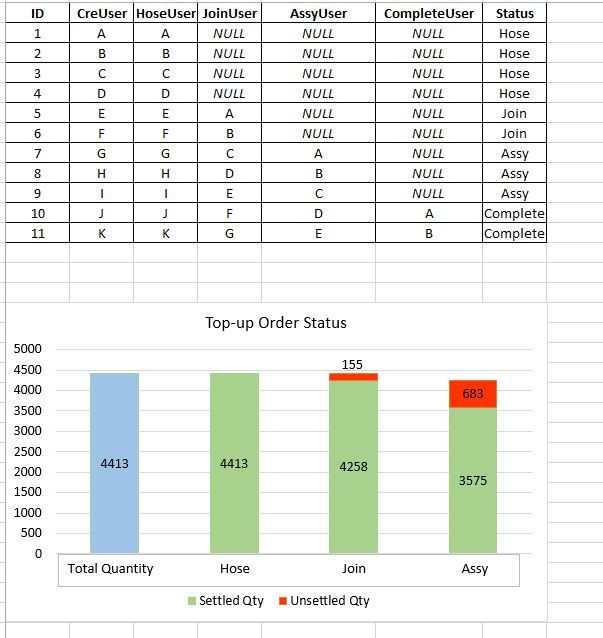Hi, I've been using Qlik Sense for about one month and recently I've encountered a problem which I've been trying to solve since last week but until now still couldn't, so I really hope someone can help me here.

So I have this data shown in the picture above with 7 columns, and I need to get a graph as shown. The total quantity can be obtained from the CreUser data, and for the Hose, Join, Assy, if there is data for that particular field, then it is considered settled for that field. If it is NULL, then it means unsettled for that particular field. Eg: there is four NULLs in the JoinUser column and another 7 rows with data. Hence, in the graph, the settle quantity for Join field is 7 and unsettled is 4.  In the end, the sum of settle and unsettle qty for all the fields must be equal to the total quantity.

What I've done is I created another four new columns in qlik sense to input '0' and '1' to indicated the presence of data in those four fields (Hose, Join, Assy, Complete), and then i concatenate the data, for eg: 1-0-0-0. For the process, it is for sure that they will start from Hose, then go to Join, then Assy and finally complete. So according to binary number, the possibilities will only be 1-0-0-0, 1-1-0-0, 1-1-1-0, 1-1-1-1. I've tried using all the commands that i found from the internet until I'm confused myself but I still can't get the graph out. Can someone help me? Thank you in advance !!!

Labels (1)
• Qlik Sense

1 Solution

Accepted Solutions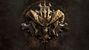Contributor II

Hello Yean,

Not a easy solution but I've found a way to display what you seek!

So for each field, you should have a key, like this:

*,
If(ISNULL(HoseUser),0 , 1) AS HoseKey,
If(ISNULL(JoinUser),0 , 1) AS JoinKey,
If(ISNULL(AssyUser),0 , 1) AS AssyKey,
If(ISNULL(CompleteUser),0 , 1) AS CompleteKey

And then in the Dimension field of your visualization:

ValueList('Total Quantity','Hose','Join','Assy','Complete')

2 Measures:

Settled Qty:

If(ValueList('Total Quantity','Hose','Join','Assy','Complete') = 'Total Quantity',
Count(ID),
If(ValueList('Total Quantity','Hose','Join','Assy','Complete') = 'Hose',
Count({<HoseKey = {'1'}>} ID),
If(ValueList('Total Quantity','Hose','Join','Assy','Complete') = 'Join',
Count({<JoinKey = {'1'}>} ID),
If(ValueList('Total Quantity','Hose','Join','Assy','Complete') = 'Assy',
Count({<AssyKey = {'1'}>} ID),
If(ValueList('Total Quantity','Hose','Join','Assy','Complete') = 'Complete',
Count({<CompleteKey = {'1'}>} ID),
)
)
)
)
)

and

Unsettled Qty:

If(ValueList('Total Quantity','Hose','Join','Assy','Complete') = 'Hose',
Count({<HoseKey = {'0'}>} ID),
If(ValueList('Total Quantity','Hose','Join','Assy','Complete') = 'Join',
Count({<JoinKey = {'0'}>} ID),
If(ValueList('Total Quantity','Hose','Join','Assy','Complete') = 'Assy',
Count({<AssyKey = {'0'}>} ID),
If(ValueList('Total Quantity','Hose','Join','Assy','Complete') = 'Complete',
Count({<CompleteKey = {'0'}>} ID),
)
)
)
)

There you go!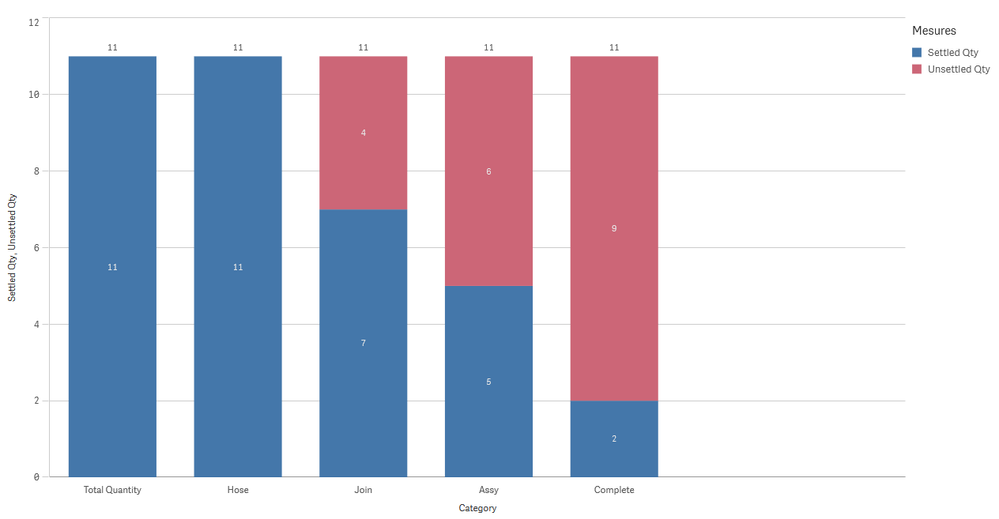4 RepliesContributor II

Hello Yean,

Not a easy solution but I've found a way to display what you seek!

So for each field, you should have a key, like this:

*,
If(ISNULL(HoseUser),0 , 1) AS HoseKey,
If(ISNULL(JoinUser),0 , 1) AS JoinKey,
If(ISNULL(AssyUser),0 , 1) AS AssyKey,
If(ISNULL(CompleteUser),0 , 1) AS CompleteKey

And then in the Dimension field of your visualization:

ValueList('Total Quantity','Hose','Join','Assy','Complete')

2 Measures:

Settled Qty:

If(ValueList('Total Quantity','Hose','Join','Assy','Complete') = 'Total Quantity',
Count(ID),
If(ValueList('Total Quantity','Hose','Join','Assy','Complete') = 'Hose',
Count({<HoseKey = {'1'}>} ID),
If(ValueList('Total Quantity','Hose','Join','Assy','Complete') = 'Join',
Count({<JoinKey = {'1'}>} ID),
If(ValueList('Total Quantity','Hose','Join','Assy','Complete') = 'Assy',
Count({<AssyKey = {'1'}>} ID),
If(ValueList('Total Quantity','Hose','Join','Assy','Complete') = 'Complete',
Count({<CompleteKey = {'1'}>} ID),
)
)
)
)
)

and

Unsettled Qty:

If(ValueList('Total Quantity','Hose','Join','Assy','Complete') = 'Hose',
Count({<HoseKey = {'0'}>} ID),
If(ValueList('Total Quantity','Hose','Join','Assy','Complete') = 'Join',
Count({<JoinKey = {'0'}>} ID),
If(ValueList('Total Quantity','Hose','Join','Assy','Complete') = 'Assy',
Count({<AssyKey = {'0'}>} ID),
If(ValueList('Total Quantity','Hose','Join','Assy','Complete') = 'Complete',
Count({<CompleteKey = {'0'}>} ID),
)
)
)
)

There you go!Contributor
Author

I tried your solution and I really got the graph that I wanted. However, I wanna ask for the dimension, is it a must to use the valuelist()? Is there a way to get the graph by using the data from the table for the dimension? Because besides the status, I still need to show other alternative dimensions, for eg: by YearMonth and by Machine. If I use the valuelist function, then my YearMonth and Machine wouldn't show out. Sorry I forgot to include the column of Date and Machines in the example table above.Contributor II

You're really lucky, I just did something similar in the last few weeks. 😁

The problem is that you want results for different fields grouped in the same dimension.

TableTemp:
CrossTable(typeUser, Settled, 2)
ID,
Status,
CreUser,
If(ISNULL(HoseUser), 0, HoseUser) AS HoseUser,
If(ISNULL(JoinUser), 0, HoseUser) AS JoinUser,
If(ISNULL(AssyUser), 0, HoseUser) AS AssyUser,
If(ISNULL(CompleteUser), 0, HoseUser) AS CompleteUser

This will transform your 5 fields in 1 so you'll get something like that: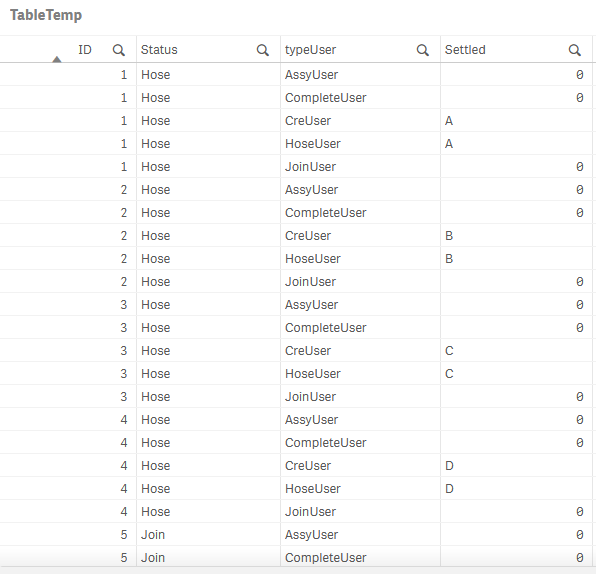And then you can add your new field "typeUser" as a dimension
With the following measures

Settled Qty:

Count(distinct {<Settled -= {'0'}>} ID)

Unsettled Qty:

Count(distinct {<Settled = {'0'}>} ID)

This way, you should be able to add alternative dimension without any issue!Contributor
Author

Thank you very much !!!! I've tried using the crosstable and I got it. But since I was told not to unlock the script for the published version, so I'll just mark the previous one as the solution. For the previous solution, I was able to show the YearMonth by using the filter as well. Anyway, really thanks a lot for your help !!!Tags
Community Browser